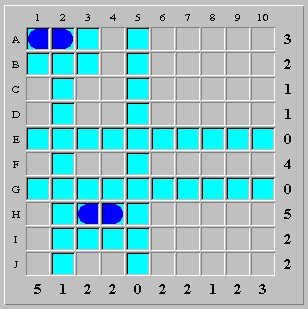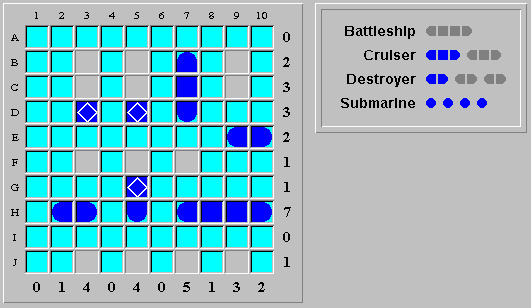Solver Rule: Common Ship Segments Found

There are several candidate positions for the ship, and the positions overlap each other. In this case, the overlapped squares are ship segments (no matter which position is the correct one), allowing you to finalize the overlapped squares as segment wildcards.

ExampleThe battleship can be placed in two possible positions only: (F,6)-(F,9) or (F,7)-(F,10). In both cases, the squares (F,7), (F,8) and (F,9) are ship segments, allowing you to finalize these three squares as segment wildcards.

There is an added "bonus" in the above case: because row F has a tally of four and the battleship is confined to the squares (F,6)-(F,10), the other unknown squares along the row (i.e. (F,1), (F,3) and (F,4)) must be water.

Example (virtual ships)The above board has two virtual destroyers remaining (one cruiser and one destroyer). There are four valid virtual destroyer positions:

À (B,3) - (C,3)

À (C,3) - (D,3)

À (F,5) - (G,5)

À (G,5) - (H,5)

(The destroyer position (C,5)-(D,5) is not valid, as it would result in four destroyers on the board).

In how many ways can the two remaining virtual destroyers be placed on the board? Here are the three valid ways to place them:

À (B,3)-(C,3) and (G,5)-(H,5)

À (C,3)-(D,3) and (F,5)-(G,5)

À (C,3)-(D,3) and (G,5)-(H,5)

It is obvious that a virtual destroyer must be placed in either (B,3)-(C,3) or (C,3)-(D,3). Since the square (C,3) is common to both positions, (C,3) must be a ship segment.